﻿ 5 BEST SHORTCUT TECHNIQUES OF MULTIPLICATION IN VEDIC MATHEMATICS #EDUCRATSWEB
Guest Post | Submit   Job information   Contents   Link   Youtube Video   Photo   Practice Set   Affiliated LinkRegisterLoginJoin Our Telegram Group https://t.me/educratsweb

### 5 Best Shortcut Techniques of Multiplication in Vedic Mathematics

Posted By educratsweb.comStudy Material 🗓 Sunday November 17 2019 👁 133Tirthaji Maharaj has classified tricks to Multiply Numbers in Vedic Mathematics in Specific and General Methods. Specific Multiplication Methods can be applied when numbers satisfy certain conditions like both numbers closer to 100 or numbers closer to each other or addition of last digits of both numbers is 10, etc. While General Multiplication Methods can be applied to any types of numbers.

Depending on Specific and General Techniques, Multiplication in Vedic Mathematics are classified in the form of Sutras as below. Lets see the  Vedic Mathematics Multiplication techniques.

1. Nikhilam Sutra (Specific Technique)
2. Anurupyena Sutra (Specific Technique)
3. Urdhva Tiryak Sutra and Vinculum Process (General Technique)
4. Ekayunena Purvena (Specific Technique)

## Nikhilam Sutra:

This is most simplest trick to multiply numbers using Vedic Mathematics. I personally like this method a lot as multiplication can be done in mind as well.

Using Nikhilam Sutra it is simpler to multiply numbers like 98 & 95, 997 & 987, 102 & 112, 995 & 1008 i.e. the numbers which are closer to power of 10.  This Sutra is a Specific method of Multiplication in Vedic Mathematics which shows shortcuts to multiply numbers which are closer to power of 10 (10, 100, 1000, etc. )

This will generate 3 cases:

• Numbers closer and less than power of 10. Example: 97 * 96, 994 * 992, etc
• Numbers closer and greater than power of 10. Example: 102* 108, 1004 * 1012, etc
• Numbers closer and lying on both sides of power of 10. Example: 102* 95, 1004 * 991, etc

Let’s see few examples on this:## Anurupyena Sutra:

This is a sub-type of Nikhilam Sutra and another vedic math multiplication trick when numbers are not closer to power of 10 but are closer to themselves. It works on concept of Working Base and then apply Nikhilam Sutra.

For Example – Multiplication of Numbers like  63 & 67.

Process:

1. Working Base(W.B.) concept: As the numbers (63 & 67) are closer to 60, we take working base as 60 (6*10) instead of 100, here factor is 6.
2. Apply concept of Nikhilam as discussed previously i.e. 63 is 3 greater than 60 and 67 is 7 greater than 60
3. Multiply 3 and 7 to get 21 in 2nd compartment. As base is *10, thus we need to have only 1 digit in 2nd compartment and hence need to carry forward 2 to 1st compartment.
4. Like Nikhilam Sutra, Cross Addition of 63 & 7 or 67 & 3 gives 70.
5. In Anurupyena Sutra, before adding carry forward directly to 1st compartment we need to multiply by the factor (6) and then add the carry forward. This Carry Forward (2) is added to 420

Same multiplication 63 and 67 can be solved by considering Working Base of 70 (10 * 7) as below.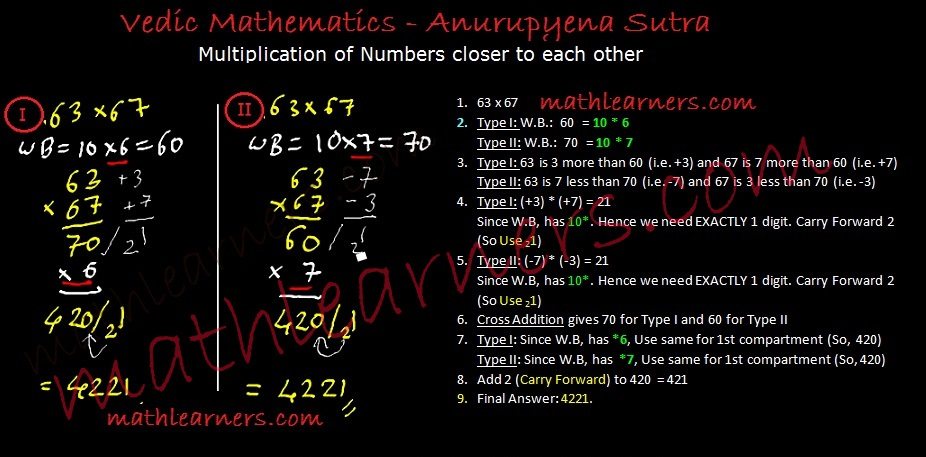Click Here  To understand the Process and More Examples of Anurupyena Sutra

## Urdhva Tiryak Sutra:

This is another great shortcut method of multiplication using Vedic Mathematics. Urdhva Tiryak is General method of multiplication in Vedic Maths which provides shortcut to multiply any types of numbers.

It can be applied very easily to multiply 3 digit numbers, multiply 4 digits numbers and even more than 4 digit numbers.

Lets see an example Multiplication of 3 digit numbers:

Formula Used:  (ax2+bx+c)(dx2+ex+f) = adx4 + (ae+bd)x3 + (af+be+cd)x2 + (bf+ce)x + cf

Process: (Left –> Right)1. Vertical Multiplication of 1st digits of 2 numbers.
2. Crosswise Multiplication Addition of 1st 2 digits 2 numbers. (i.e. Crosswise Multiplication of 1st 2 digits and adding them.)
3. Crosswise Multiplication Addition of all 3 digits of both the numbers.
4. Crosswise Multiplication Addition of last 2 digits 2 numbers.
5. Vertical Multiplication of last digits 2 numbers.
6. For all steps, except 1st step, each compartment needs to have ONLY 1 digits. If not then carry forward initial digits to previous compartment (Check below examples to understand).Click Here to Check Process, Multiplication of 4 & more examples using UrdhvaTiryak Sutra.

## Vinculum Process of Multiplication: �

Vinculum is a special method of Vedic Maths Multiplication which is used with Urdhva Tiryak whenever we have bigger digits like 6,7,8 and 9.

Vinculum is a process applied when numbers have bigger digits like 6,7,8,9. Carrying out operations like multiplication with bigger digits is time consuming and little tougher as compared to smaller digits. Hence such digits 6,7,8 and 9 are converted to smaller digits like 4,3,2 and 1 using Vinculum Process.

I highly recommend to go through the� concept of Vinculum Process.## Ekayunena Purvena Sutra:

This sutra is applicable whenever multiplier has only 9’s as digits.

Example: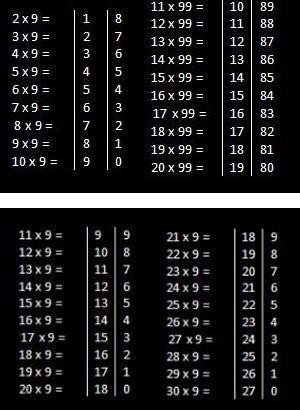Click Here => To understand the Process and more examples on Ekayunena Purvena Sutra.

## Antyaordasake’pi:

This sutra has another great multiplication trick in Vedic Mathematics which can be applied when last digits of both numbers totals as 10.

Steps:

1. Check if addition of last digits of the numbers is 10.
2. If yes, multiply them and write in 2nd compartment.
3. Apply Ekadhikena Purvena for the remaining digits i.e. Add 1 to the remaining digits.
4. Eg: In case of 34 x 36, Apply Ekadhikena Purvena on 3 so we have 4. Now multiply 3 and 4 and write in the 1st compartment.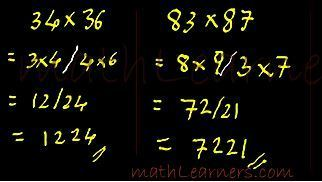Contents shared By educratsweb.com
if you have any information regarding Job, Study Material or any other information related to career. you can Post your article on our website. Click here to Register & Share your contents.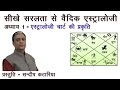Hindi Learn Vedic Astrology Lesson 1 by Sundeep KatariaLearn Vedic Astrology Step by Step Lesson 1 by Sundeep Katariaबिना पेन उठाये Calculation कैसे करे (Vedic Maths, Digit Sum) for SSC, BANK, CATकार्यपालक सहायक परीक्षा (Executive Assistant Exam) सफलता के टिप्स नंबर 1 | Shortcut Key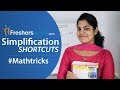Aptitude made easy- Simplification Shortcuts 1 - SSC,Railway,IBPS #MathtricksSales Motivational Video Sales Training & Techniques in Hindi by Vivek Bindra